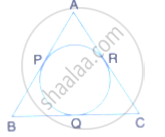Share

From the Given Figure, Prove That: Ap + Bq + Cr = Bp + Cq + Ar Also Show That: Ap + Bq + Cr = 1/2× Perimeter of δAbc. - ICSE Class 10 - Mathematics

ConceptTangent Properties - If a Line Touches a Circle and from the Point of Contact, a Chord is Drawn, the Angles Between the Tangent and the Chord Are Respectively Equal to the Angles in the Corresponding Alternate Segments

Question

From the given figure, prove that:
AP + BQ + CR = BP + CQ + ARAlso show that:
AP + BQ + CR = 1/2× Perimeter of ΔABC.

Solution

Since from B, BQ and BP are the tangents to the circle
Therefore, BQ = BP ………..(i)
Similarly, we can prove that
AP = AR …………..(ii)
and CR = CQ ………(iii)
AP + BQ + CR = BP + CQ + AR ………(iv)
Adding AP + BQ + CR to both sides
2(AP + BQ + CR) = AP + PQ + CQ + QB + AR + CR
2(AP + BQ + CR) = AB + BC + CA
Therefore, AP + BQ + CR = 1/2× (AB + BC + CA)
AP + BQ + CR = 1/2× perimeter of triangle ABC

Is there an error in this question or solution?

Video TutorialsVIEW ALL 

Solution From the Given Figure, Prove That: Ap + Bq + Cr = Bp + Cq + Ar Also Show That: Ap + Bq + Cr = 1/2× Perimeter of δAbc. Concept: Tangent Properties - If a Line Touches a Circle and from the Point of Contact, a Chord is Drawn, the Angles Between the Tangent and the Chord Are Respectively Equal to the Angles in the Corresponding Alternate Segments.
S